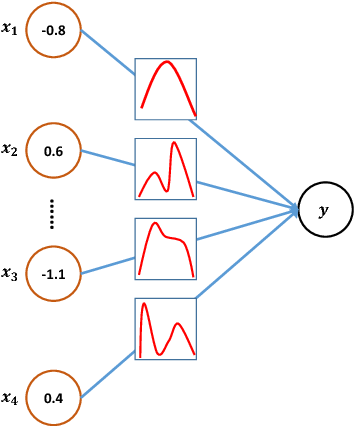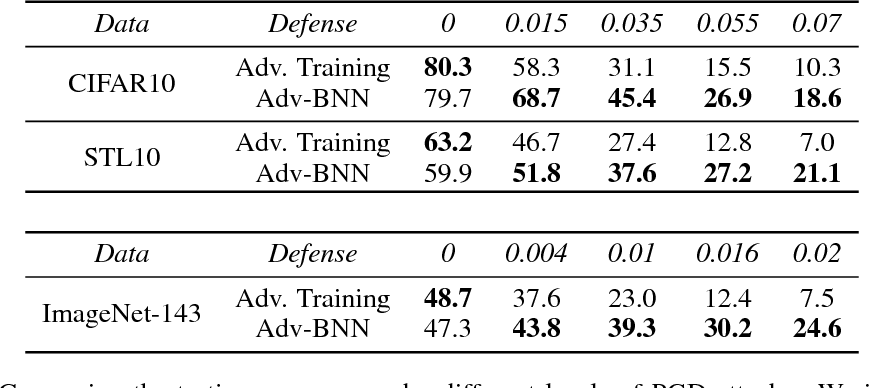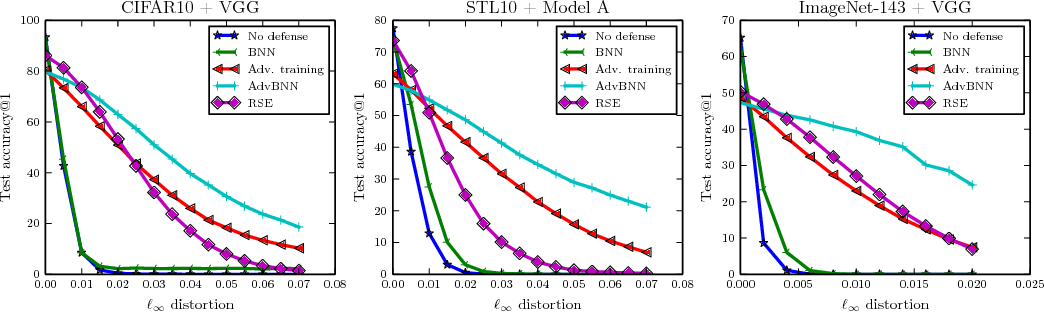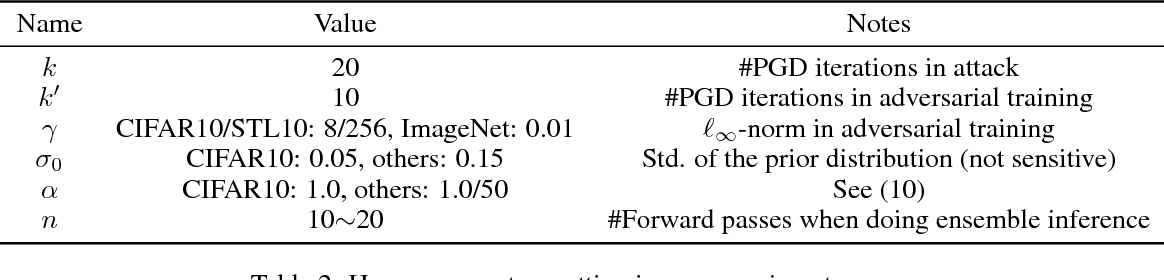We present a new algorithm to train a robust neural network against adversarial attacks. Our algorithm is motivated by the following two ideas. First, although recent work has demonstrated that fusing randomness can improve the robustness of neural networks (Liu 2017), we noticed that adding noise blindly to all the layers is not the optimal way to incorporate randomness. Instead, we model randomness under the framework of Bayesian Neural Network (BNN) to formally learn the posterior distribution of models in a scalable way. Second, we formulate the mini-max problem in BNN to learn the best model distribution under adversarial attacks, leading to an adversarial-trained Bayesian neural net. Experiment results demonstrate that the proposed algorithm achieves state-of-the-art performance under strong attacks. On CIFAR-10 with VGG network, our model leads to 14\% accuracy improvement compared with adversarial training (Madry 2017) and random self-ensemble (Liu 2017) under PGD attack with $0.035$ distortion, and the gap becomes even larger on a subset of ImageNet.

* Code will be made available at https://github.com/xuanqing94/BayesianDefense
Click to Read Paper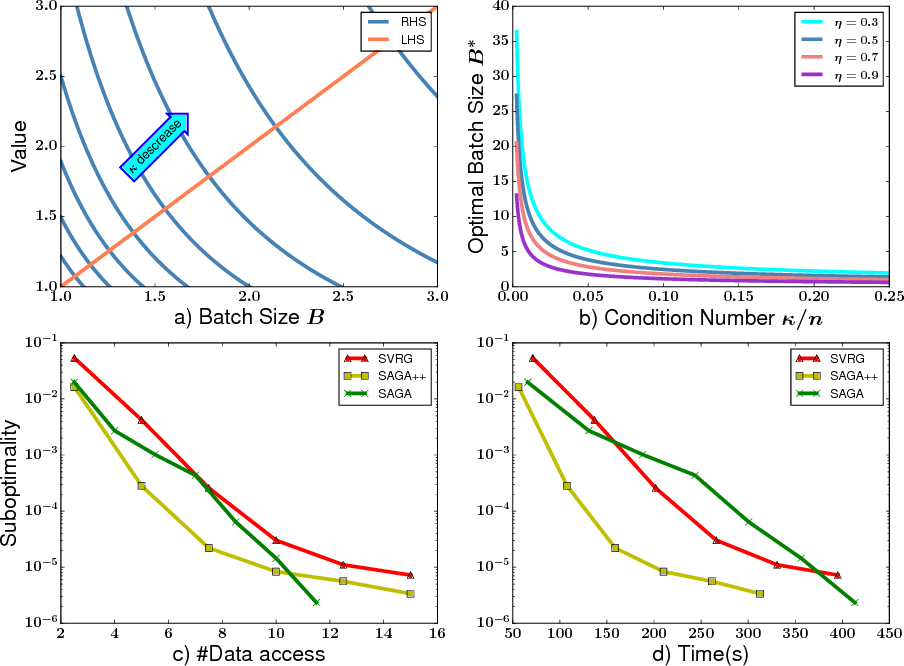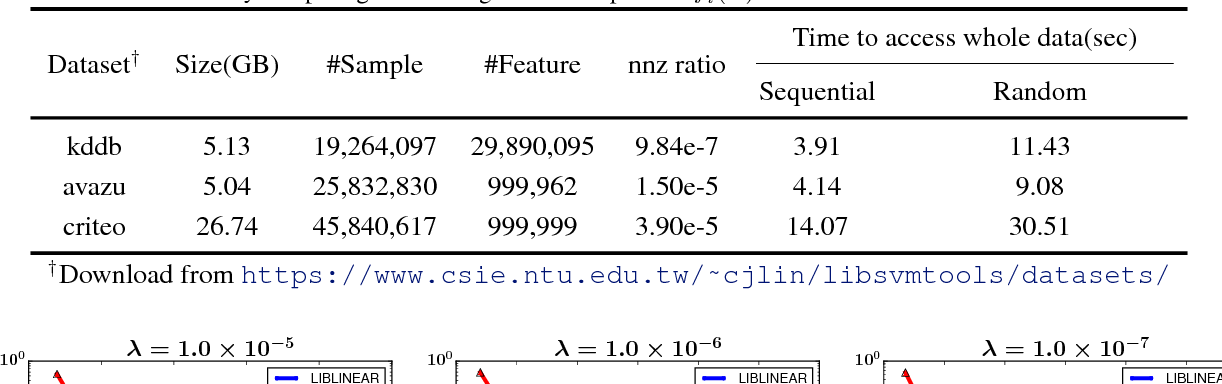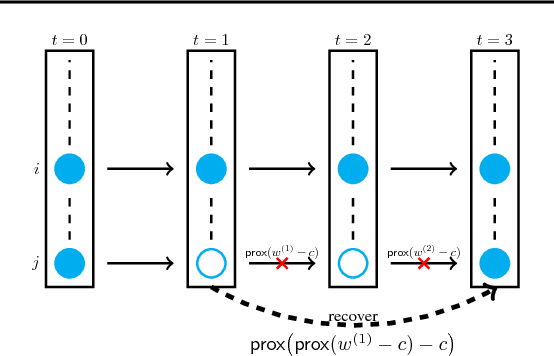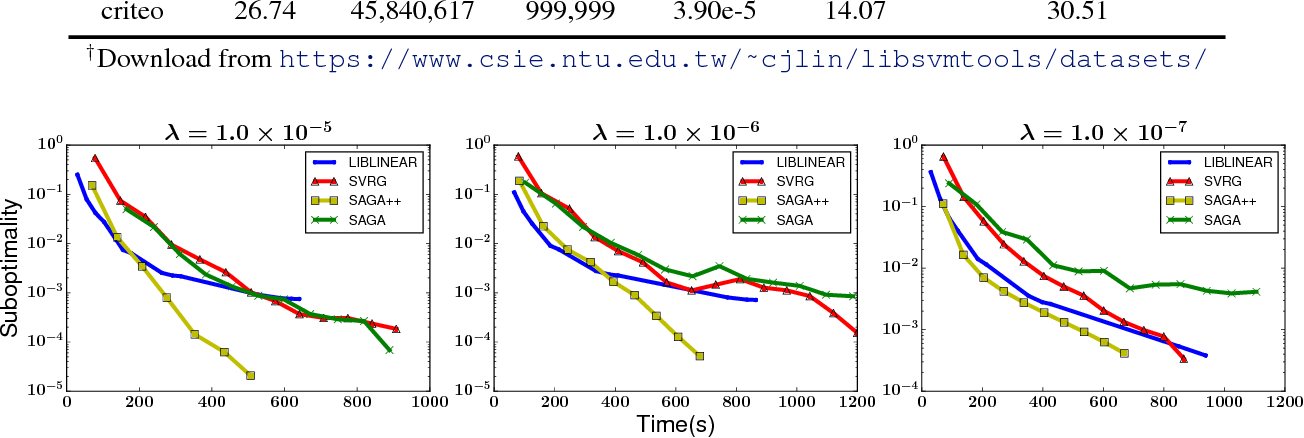In this paper we study a family of variance reduction methods with randomized batch size---at each step, the algorithm first randomly chooses the batch size and then selects a batch of samples to conduct a variance-reduced stochastic update. We give the linear convergence rate for this framework for composite functions, and show that the optimal strategy to achieve the optimal convergence rate per data access is to always choose batch size of 1, which is equivalent to the SAGA algorithm. However, due to the presence of cache/disk IO effect in computer architecture, the number of data access cannot reflect the running time because of 1) random memory access is much slower than sequential access, 2) when data is too big to fit into memory, disk seeking takes even longer time. After taking these into account, choosing batch size of $1$ is no longer optimal, so we propose a new algorithm called SAGA++ and show how to calculate the optimal average batch size theoretically. Our algorithm outperforms SAGA and other existing batched and stochastic solvers on real datasets. In addition, we also conduct a precise analysis to compare different update rules for variance reduction methods, showing that SAGA++ converges faster than SVRG in theory.

Click to Read Paper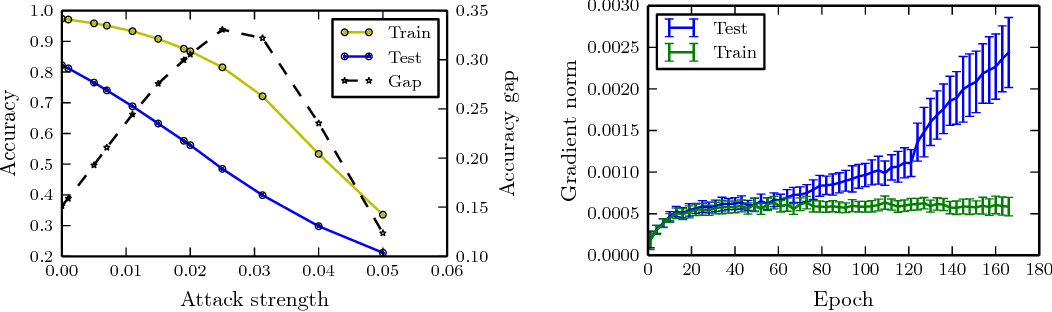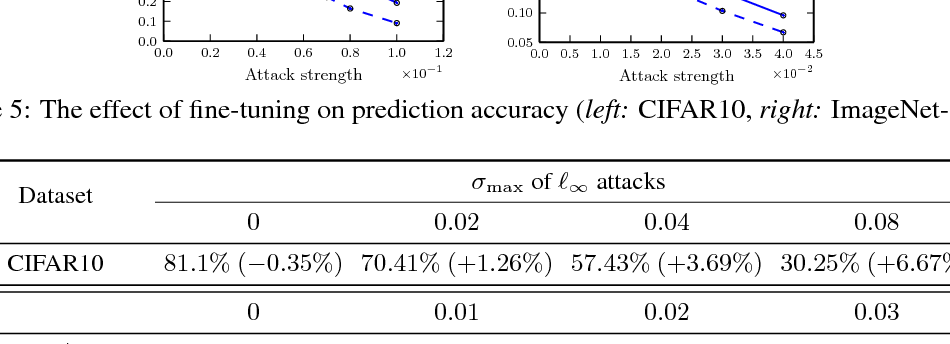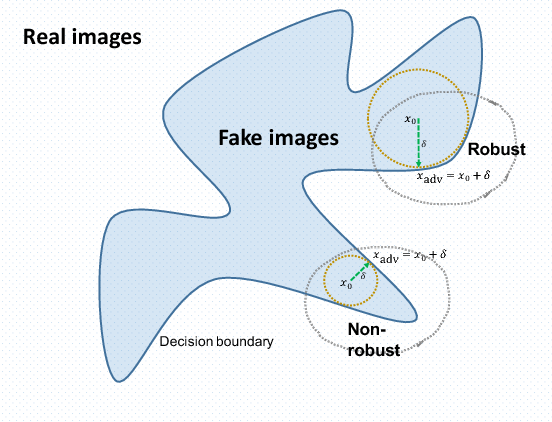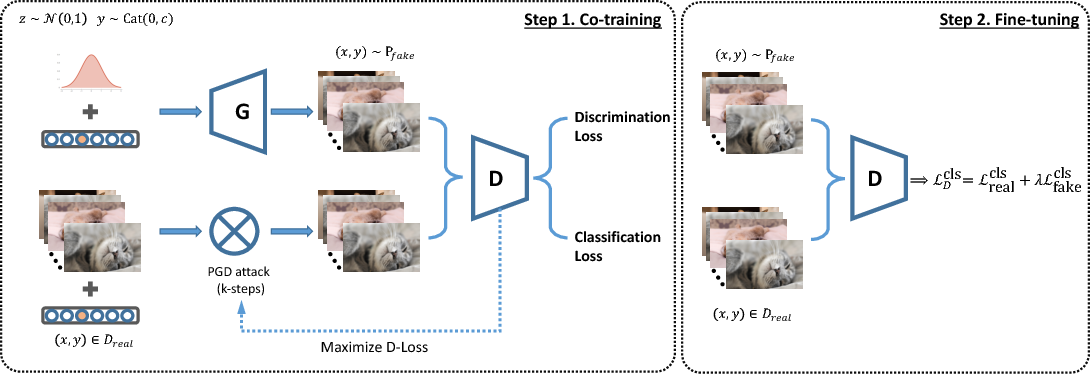* NIPS 2018 submission, under review. v2: More experiments on comparing inception score, release code and some minor fixes
Click to Read Paper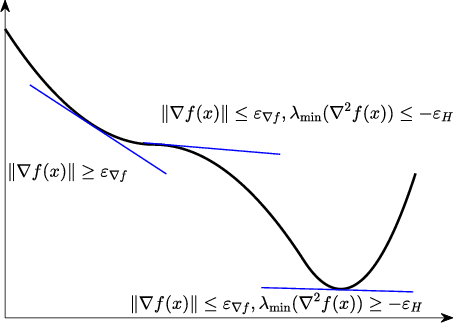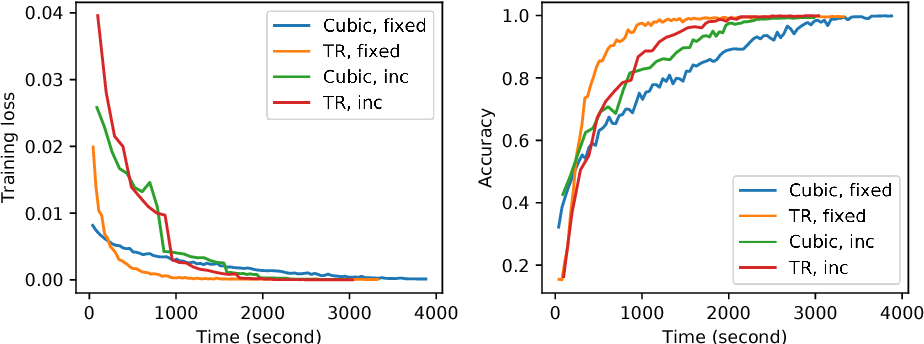Trust region and cubic regularization methods have demonstrated good performance in small scale non-convex optimization, showing the ability to escape from saddle points. Each iteration of these methods involves computation of gradient, Hessian and function value in order to obtain the search direction and adjust the radius or cubic regularization parameter. However, exactly computing those quantities are too expensive in large-scale problems such as training deep networks. In this paper, we study a family of stochastic trust region and cubic regularization methods when gradient, Hessian and function values are computed inexactly, and show the iteration complexity to achieve $\epsilon$-approximate second-order optimality is in the same order with previous work for which gradient and function values are computed exactly. The mild conditions on inexactness can be achieved in finite-sum minimization using random sampling. We show the algorithm performs well on training convolutional neural networks compared with previous second-order methods.

Click to Read Paper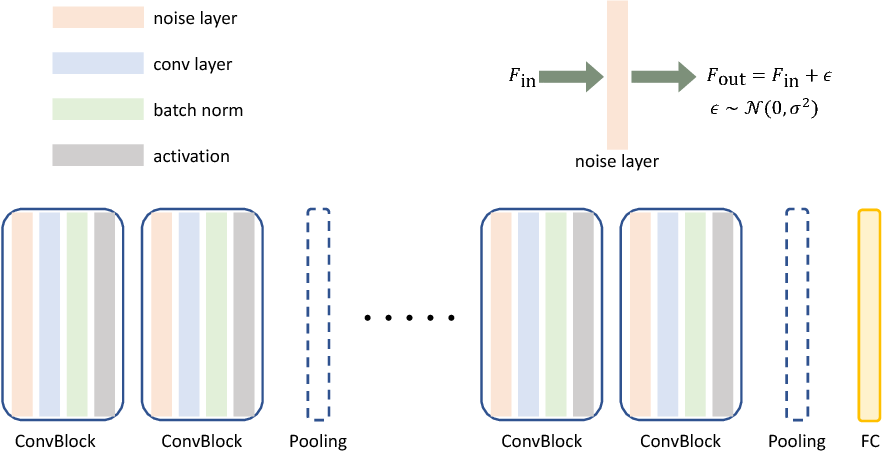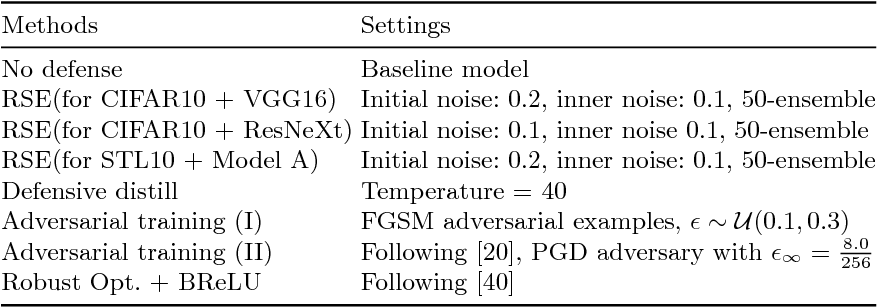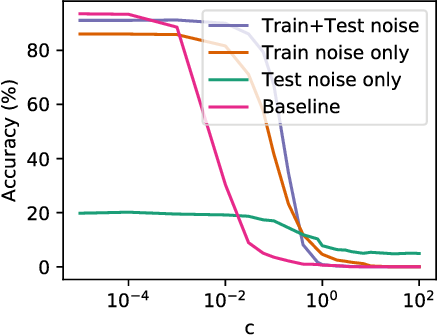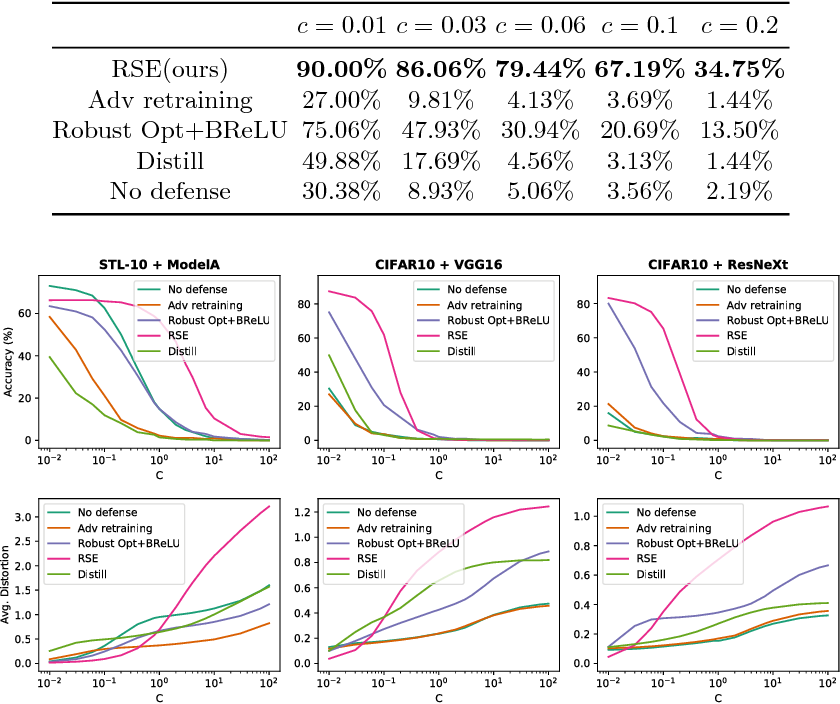Recent studies have revealed the vulnerability of deep neural networks: A small adversarial perturbation that is imperceptible to human can easily make a well-trained deep neural network misclassify. This makes it unsafe to apply neural networks in security-critical applications. In this paper, we propose a new defense algorithm called Random Self-Ensemble (RSE) by combining two important concepts: {\bf randomness} and {\bf ensemble}. To protect a targeted model, RSE adds random noise layers to the neural network to prevent the strong gradient-based attacks, and ensembles the prediction over random noises to stabilize the performance. We show that our algorithm is equivalent to ensemble an infinite number of noisy models $f_\epsilon$ without any additional memory overhead, and the proposed training procedure based on noisy stochastic gradient descent can ensure the ensemble model has a good predictive capability. Our algorithm significantly outperforms previous defense techniques on real data sets. For instance, on CIFAR-10 with VGG network (which has 92\% accuracy without any attack), under the strong C\&W attack within a certain distortion tolerance, the accuracy of unprotected model drops to less than 10\%, the best previous defense technique has $48\%$ accuracy, while our method still has $86\%$ prediction accuracy under the same level of attack. Finally, our method is simple and easy to integrate into any neural network.

* ECCV 2018 camera ready
Click to Read Paper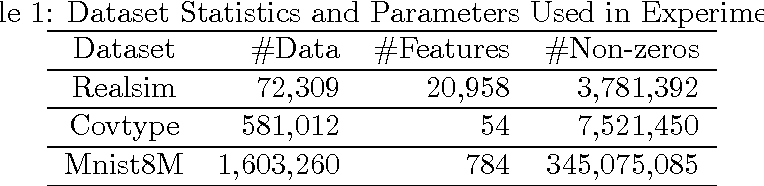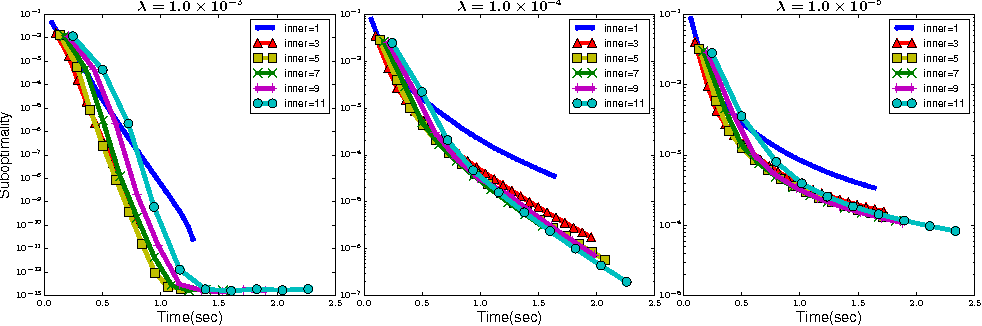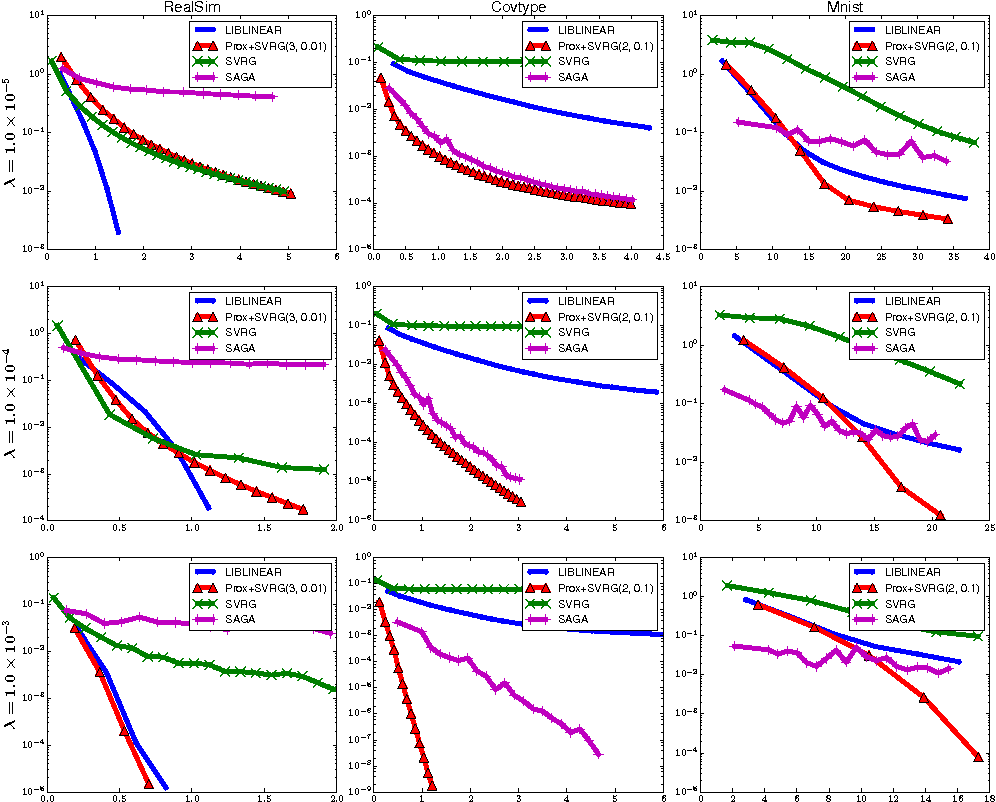We propose a fast proximal Newton-type algorithm for minimizing regularized finite sums that returns an $\epsilon$-suboptimal point in $\tilde{\mathcal{O}}(d(n + \sqrt{\kappa d})\log(\frac{1}{\epsilon}))$ FLOPS, where $n$ is number of samples, $d$ is feature dimension, and $\kappa$ is the condition number. As long as $n > d$, the proposed method is more efficient than state-of-the-art accelerated stochastic first-order methods for non-smooth regularizers which requires $\tilde{\mathcal{O}}(d(n + \sqrt{\kappa n})\log(\frac{1}{\epsilon}))$ FLOPS. The key idea is to form the subsampled Newton subproblem in a way that preserves the finite sum structure of the objective, thereby allowing us to leverage recent developments in stochastic first-order methods to solve the subproblem. Experimental results verify that the proposed algorithm outperforms previous algorithms for $\ell_1$-regularized logistic regression on real datasets.

Click to Read Paper